8.11.1.3

### 3Special Functions 🔗 ℹ

 (require math/special-functions) package: math-lib

The term “special function” has no formal definition. However, for the purposes of the math library, a special function is one that is not elementary.

The special functions are split into two groups: Real Functions and Flonum Functions. Functions that accept real arguments are usually defined in terms of their flonum counterparts, but are different in two crucial ways:
• Many return exact values for certain exact arguments.

• When applied to exact arguments outside their domains, they raise an exn:fail:contract instead of returning +nan.0.

Currently, math/special-functions does not export any functions that accept or return complex numbers. Mathematically, some of them could return complex numbers given real numbers, such hurwitz-zeta when given a negative second argument. In these cases, they raise an exn:fail:contract (for an exact argument) or return +nan.0 (for an inexact argument).

Most real functions have more than one type, but they are documented as having only one. The documented type is the most general type, which is used to generate a contract for uses in untyped code. Use :print-type to see all of a function’s types.

A function’s types state theorems about its behavior in a way that Typed Racket can understand and check. For example, lambert has these types:
 (case-> (Zero -> Zero) (Flonum -> Flonum) (Real -> (U Zero Flonum)))

Because lambert : Zero -> Zero, Typed Racket proves during typechecking that one of its exact cases is (lambert 0) = 0.

Because the theorem lambert : Flonum -> Flonum is stated as a type and proved by typechecking, Typed Racket’s optimizer can transform the expressions around its use into bare-metal floating-point operations. For example, (+ 2.0 (lambert 3.0)) is transformed into (unsafe-fl+ 2.0 (lambert 3.0)).

The most general type Real -> (U Zero Flonum) is used to generate lambert’s contract when it is used in untyped code. Except for this discussion, this the only type documented for lambert.

#### 3.1Real Functions 🔗 ℹ

 procedure x : Real
Computes the gamma function, a generalization of the factorial function to the entire real line, except nonpositive integers. When x is an exact integer, (gamma x) is exact.

Examples:
 > (plot (list (function (λ (x) (gamma (+ 1 x))) 0 4.5 #:label "gamma(x+1)") (function (λ (x) (factorial (truncate x))) #:color 2 #:label "factorial(floor(x))")))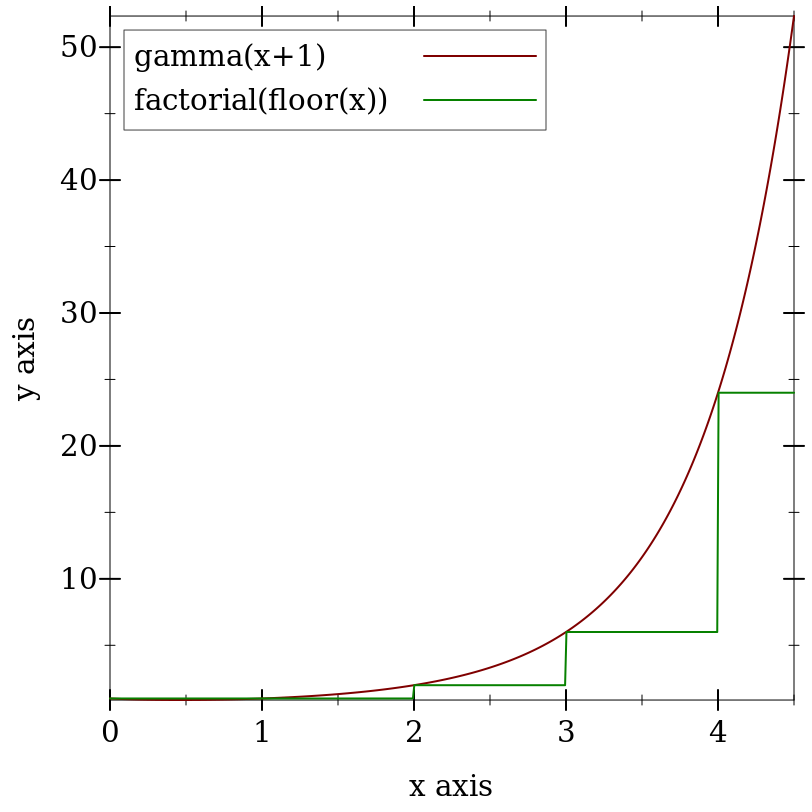> (plot (function gamma -2.5 5.5) #:y-min -50 #:y-max 50)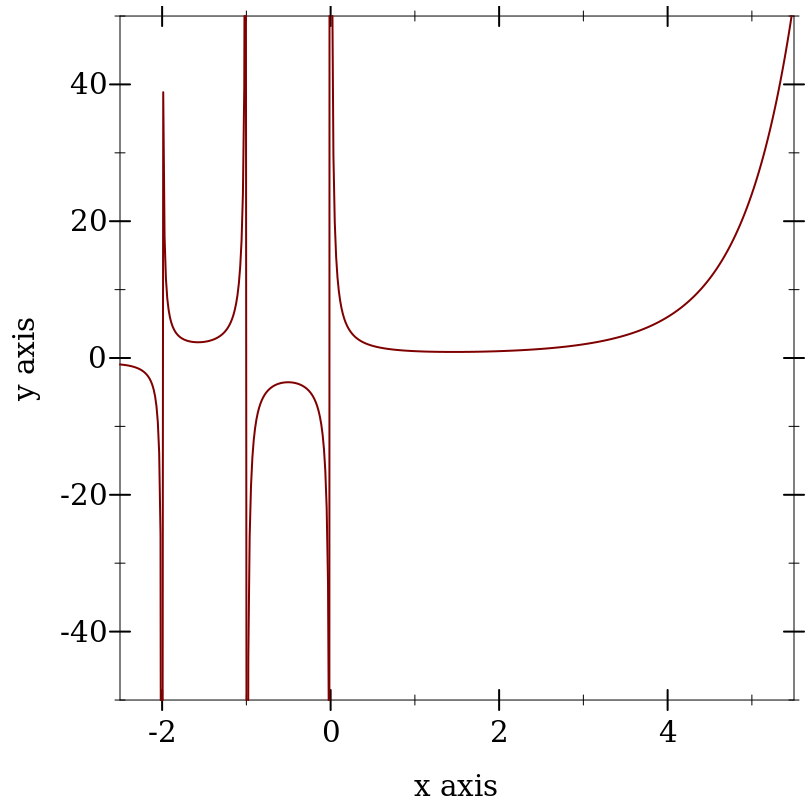> (gamma 5)

24

> (gamma 5.0)

24.0

> (factorial 4)

24

> (gamma -1)

gamma: contract violation

expected: Real, not Zero or Negative-Integer

given: -1

> (gamma -1.0)

+nan.0

> (gamma 0.0)

+inf.0

> (gamma -0.0)

-inf.0

> (gamma 172.0)

+inf.0

> (bf (gamma 172))

(bf "1.241018070217667823424840524103103992618e309")

Error is no more than 10 ulps everywhere that has been tested, and is usually no more than 4 ulps.

 procedure(log-gamma x) → (U Zero Flonum) x : Real
Like (log (abs (gamma x))), but more accurate and without unnecessary overflow. The only exact cases are (log-gamma 1) = 0 and (log-gamma 2) = 0.

Examples:
 > (plot (list (function log-gamma -5.5 10.5 #:label "log-gamma(x)") (function (λ (x) (log (abs (gamma x)))) #:color 2 #:style 'long-dash #:width 2 #:label "log(abs(gamma(x)))")))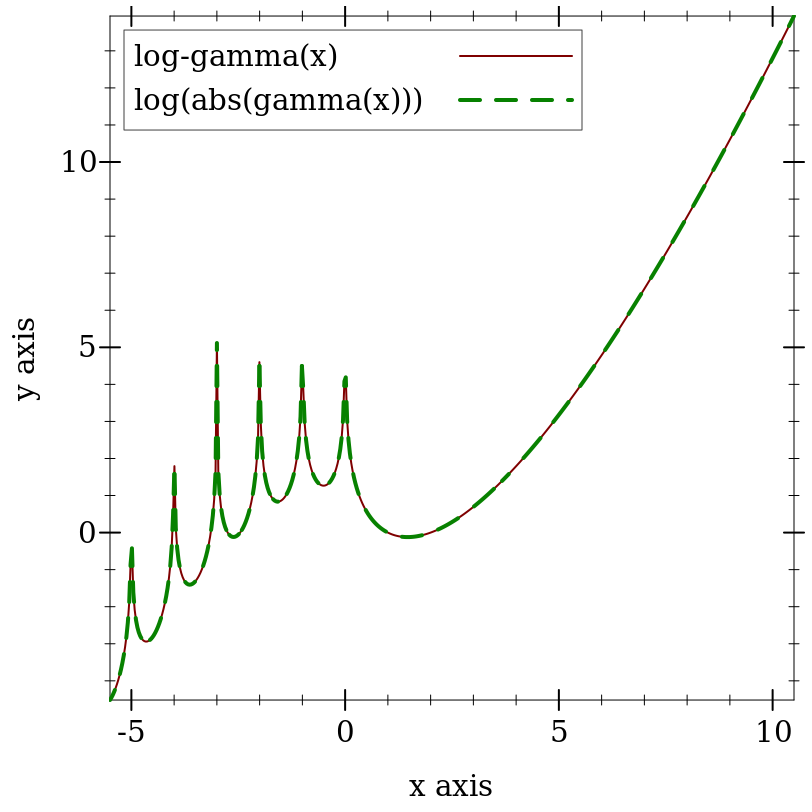> (log-gamma 5)

3.1780538303479458

> (log (abs (gamma 5)))

3.1780538303479458

> (log-gamma -1)

log-gamma: contract violation

expected: Real, not Zero or Negative-Integer

given: -1

> (log-gamma -1.0)

+inf.0

> (log-gamma 0.0)

+inf.0

> (log (abs (gamma 172.0)))

+inf.0

> (log-gamma 172.0)

711.71472580229

Error is no more than 11 ulps everywhere that has been tested, and is usually no more than 2 ulps. Error reaches its maximum near negative roots.

 procedure(psi0 x) → Flonum x : Real
Computes the digamma function, the logarithmic derivative of the gamma function.

Examples:
 > (plot (function psi0 -2.5 4.5) #:y-min -5 #:y-max 5)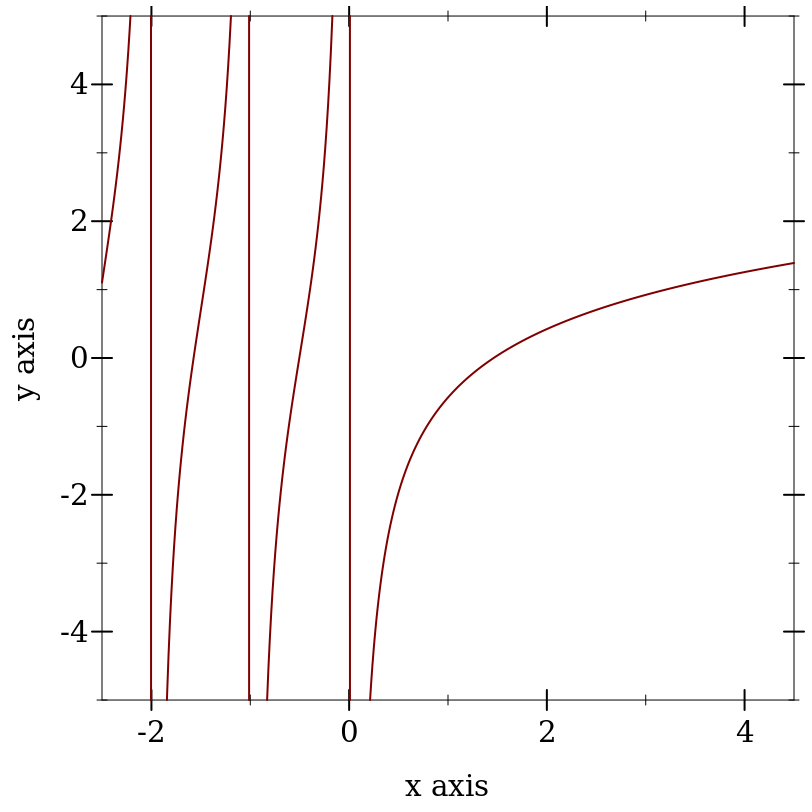> (psi0 0) psi0: contract violation expected: Real, not Zero or Negative-Integer given: 0 > (psi0 1) -0.5772156649015329 > (- gamma.0) -0.5772156649015329

Except near negative roots, maximum observed error is 2 ulps, but is usually no more than 1.

Near negative roots, which occur singly between each pair of negative integers, psi0 exhibits catastrophic cancellation from using the reflection formula, meaning that relative error is effectively unbounded. However, maximum observed absolute-error is (* 5 epsilon.0). This is the best we can do for now, because there are currently no reasonably fast algorithms for computing psi0 near negative roots with low relative error.

If you need low relative error near negative roots, use bfpsi0.

 procedure(psi m x) → Flonum m : Integer x : Real
Computes a polygamma function, or the mth logarithmic derivative of the gamma function. The order m must be a natural number, and x may not be zero or a negative integer. Note that (psi 0 x) = (psi0 x).

Examples:
 > (plot (for/list ([m  (in-range 4)]) (function (λ (x) (psi m x)) -2.5 2.5 #:color m #:style m #:label (format "psi~a(x)" m))) #:y-min -300 #:y-max 300 #:legend-anchor 'top-right)> (psi -1 2.3)

psi: contract violation

expected: Natural

given: -1

argument position: 1st

other arguments...:

2.3

> (psi 0 -1.1)

10.154163959143848

> (psi0 -1.1)

10.154163959143848

From spot checks with m > 0, error appears to be as with psi0: very low except near negative roots. Near negative roots, relative error is apparently unbounded, but absolute error is low.

 procedure(erf x) → Real x : Real
 procedure(erfc x) → Real x : Real
Compute the error function and complementary error function, respectively. The only exact cases are (erf 0) = 0 and (erfc 0) = 1.

Examples:
 > (plot (list (function erf -2 2 #:label "erf(x)") (function erfc #:color 2 #:label "erfc(x)")))> (erf 0)

0

> (erf 1)

0.8427007929497148

> (- 1 (erfc 1))

0.8427007929497148

> (erf -1)

-0.8427007929497149

> (- (erfc 1) 1)

-0.8427007929497148

Mathematically, erfc(x) = 1 - erf(x), but having separate implementations can help maintain accuracy. To compute an expression containing erf, use erf for x near 0.0. For positive x away from 0.0, manipulate (- 1.0 (erfc x)) and its surrounding expressions to avoid the subtraction:

 > (define x 5.2) > (bf-precision 128) > (define log-erf-x (bigfloat->rational (bflog (bferf (bf x))))) > (flulp-error (log (erf x)) log-erf-x) 873944876280.6095 > (flulp-error (log (- 1.0 (erfc x))) log-erf-x) 873944876280.6095 > (flulp-error (fllog1p (- (erfc x))) log-erf-x) 1.609486456125461
For negative x away from 0.0, do the same with (- (erfc (- x)) 1.0).

For erf, error is no greater than 2 ulps everywhere that has been tested, and is almost always no greater than 1. For erfc, observed error is no greater than 4 ulps, and is usually no greater than 2.

 procedure(lambert x) → (U Zero Flonum) x : Real
 procedure(lambert- x) → Flonum x : Real
Compute the Lambert W function, or the inverse of x = (* y (exp y)).

This function has two real branches. The lambert variant computes the upper branch, and is defined for x >= (- (exp -1)). The lambert- variant computes the lower branch, and is defined for negative x >= (- (exp -1)). The only exact case is (lambert 0) = 0.

Examples:
 > (plot (list (function lambert (- (exp -1)) 1) (function lambert- (- (exp -1)) -min.0 #:color 2)) #:y-min -4)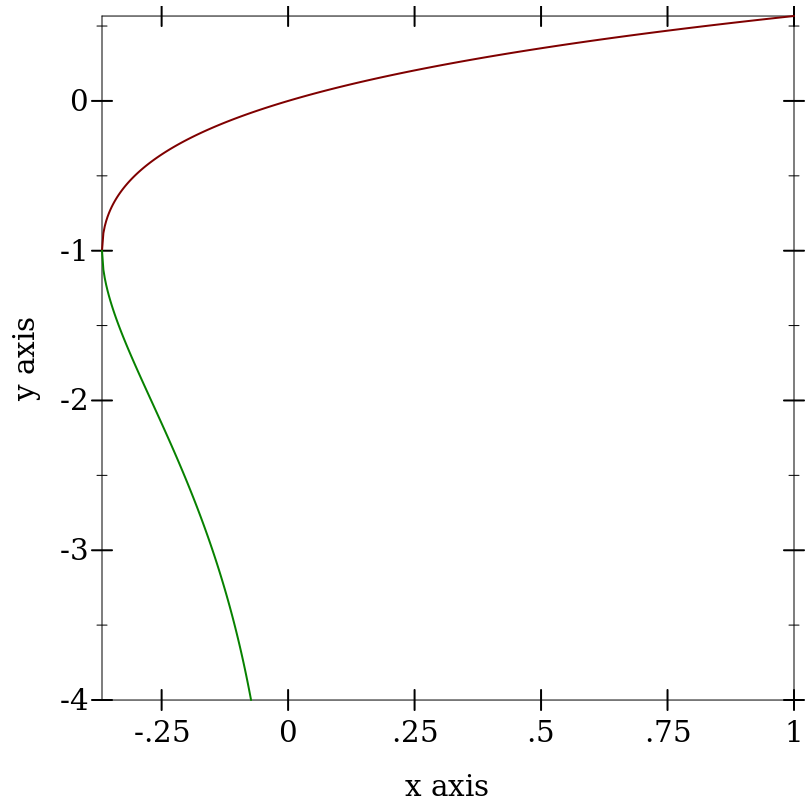> (lambert 0)

0

> (lambert (- (exp -1)))

-1.0

> (lambert -1/2)

lambert: contract violation

expected: Real >= (- (exp -1))

given: -1/2

> (lambert- 0)

lambert-: contract violation

expected: Negative-Real >= (- (exp -1))

given: 0

> (define y0 (lambert -0.1))
> (define y1 (lambert- -0.1))
> y0

-0.11183255915896297

> y1

-3.577152063957297

> (* y0 (exp y0))

-0.1

> (* y1 (exp y1))

-0.10000000000000002

The Lambert W function often appears in solutions to equations that contain n log(n), such as those that describe the running time of divide-and-conquer algorithms.

For example, suppose we have a sort that takes t = (* c n (log n)) time, and we measure the time it takes to sort an n = 10000-element list at t = 0.245 ms. Solving for c, we get
 > (define n 10000) > (define t 0.245) > (define c (/ t (* n (log n)))) > c 2.6600537016574172e-6
Now we would like to know how many elements we can sort in 100ms. We solve for n and use the solution to define a function time->sort-size:
 > (define (time->sort-size t) (exact-floor (exp (lambert (/ t c)))))
> (time->sort-size 100)

2548516

Testing the solution, we get
 > (define lst2 (build-list 2548516 values)) > (time (sort lst2 <)) cpu time: 80 real time: 93 gc time: 0

For both branches, error is no more than 2 ulps everywhere tested.

 procedure(zeta x) → Real x : Real
Computes the Riemann zeta function. If x is a nonpositive exact integer, (zeta x) is exact.

Examples:
> (plot (function zeta -2 10) #:y-min -4 #:y-max 4)> (plot (function zeta -14 -2))> (zeta 0)

-1/2

> (zeta 1)

zeta: contract violation

expected: Real, not One

given: 1

> (zeta 1.0)

-inf.0

> (zeta -1)

-1/12

> (define num 1000000)

 > (define num-coprime (for/sum ([_  (in-range num)]) (if (coprime? (random-bits 16) (random-bits 16)) 1 0)))

> (fl (/ num-coprime num))

0.607901

> (/ 1 (zeta 2))

0.6079271018540264

When s is an odd, negative exact integer, (zeta s) computes (bernoulli (- 1 s)), which can be rather slow.

Maximum observed error is 6 ulps, but is usually 3 or less.

 procedure(eta x) → Real x : Real
Computes the Dirichlet eta function. If x is a nonpositive exact integer, (eta x) is exact.

Examples:
 > (plot (function eta -10 6))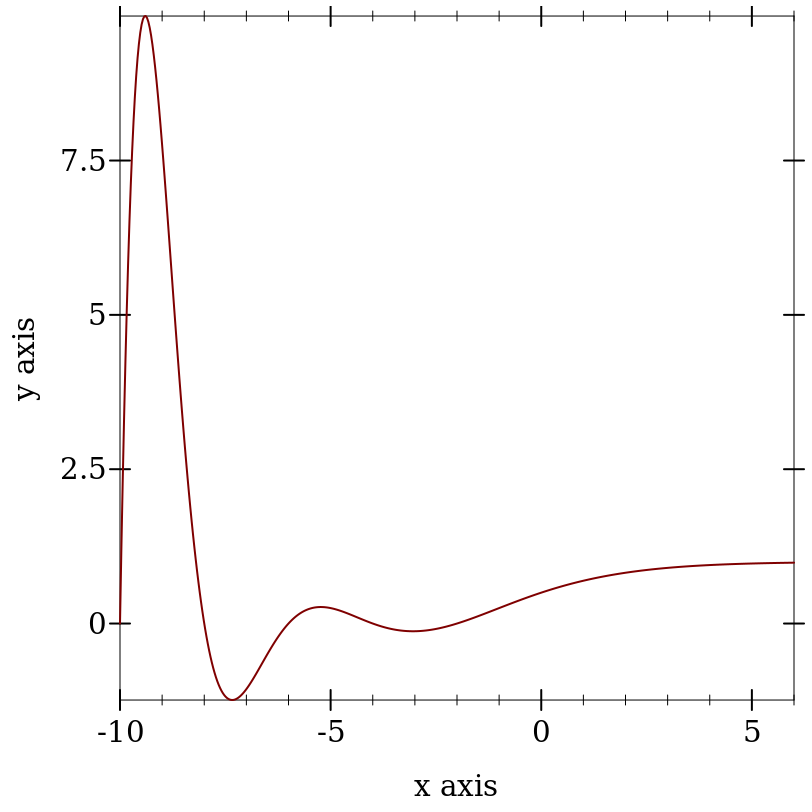> (eta 0) 1/2 > (eta -1) 1/4 > (eta 1) 0.6931471805599453 > (log 2) 0.6931471805599453

When s is an odd, negative exact integer, (eta s) computes (bernoulli (- 1 s)), which can be rather slow.

Maximum observed error is 11 ulps, but is usually 4 or less.

 procedure(hurwitz-zeta s q) → Real s : Real q : Real
Computes the Hurwitz zeta function for s > 1 and q > 0. When s = 1.0 or q = 0.0, (hurwitz-zeta s q) = +inf.0.

Examples:
 > (plot (list (function zeta 1.5 5) (function (λ (s) (hurwitz-zeta s 1)) #:color 2 #:style 'long-dash #:width 2)))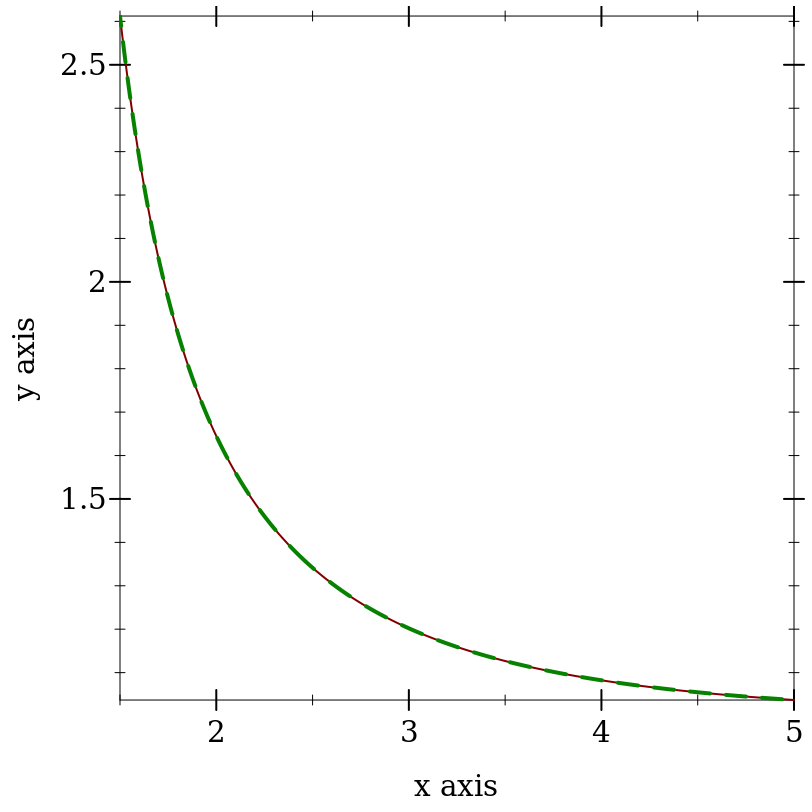> (hurwitz-zeta 1 1)

hurwitz-zeta: contract violation

expected: Real > 1

given: 1

argument position: 1st

other arguments...:

1

> (hurwitz-zeta 1.0 1.0)

+inf.0

> (hurwitz-zeta 2 1/4)

17.197329154507113

> (+ (sqr pi) (* 8 catalan.0))

17.19732915450711

While hurwitz-zeta currently raises an exception for s < 1, it may in the future return real values.

Maximum observed error is 6 ulps, but is usually 2 or less.

 procedure(beta x y) → (U Exact-Rational Flonum) x : Real y : Real
Computes the beta function for positive real x and y. Like (/ (* (gamma x) (gamma y)) (gamma (+ x y))), but more accurate.

Examples:
 > (plot3d (contour-intervals3d beta 0.25 2 0.25 2) #:angle 250)> (beta 0 0) beta: contract violation expected: positive Real given: 0 argument position: 1st other arguments...: 0 > (beta 1 5) 1/5 > (beta 1.0 5.0) 0.2

 procedure(log-beta x y) → (U Zero Flonum) x : Real y : Real
Like (log (beta x y)), but more accurate and without unnecessary overflow. The only exact case is (log-beta 1 1) = 0.

 procedure(gamma-inc k x [upper? regularized?]) → Flonum k : Real x : Real upper? : Any = #f regularized? : Any = #f
Computes the incomplete gamma integral for k > 0 and x >= 0. When upper? = #f, it integrates from zero to x; otherwise it integrates from x to infinity.

If you are doing statistical work, you should probably use gamma-dist instead, which is defined in terms of gamma-inc and is more flexible (e.g. it allows negative x).

The following identities should hold:

Examples:
 > (list (plot3d (contour-intervals3d gamma-inc 0.1 4.5 0 10) #:x-label "k" #:y-label "x" #:width 210 #:height 210) (plot3d (contour-intervals3d (λ (k x) (gamma-inc k x #t)) 0.1 4.5 0 10) #:x-label "k" #:y-label "x" #:width 210 #:height 210))

'(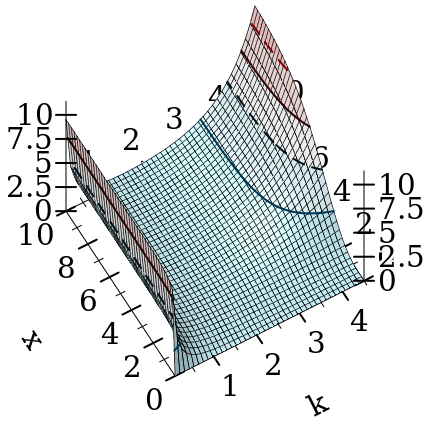)

 > (plot3d (contour-intervals3d (λ (k x) (gamma-inc k x #f #t)) 0.1 20 0 20) #:x-label "k" #:y-label "x")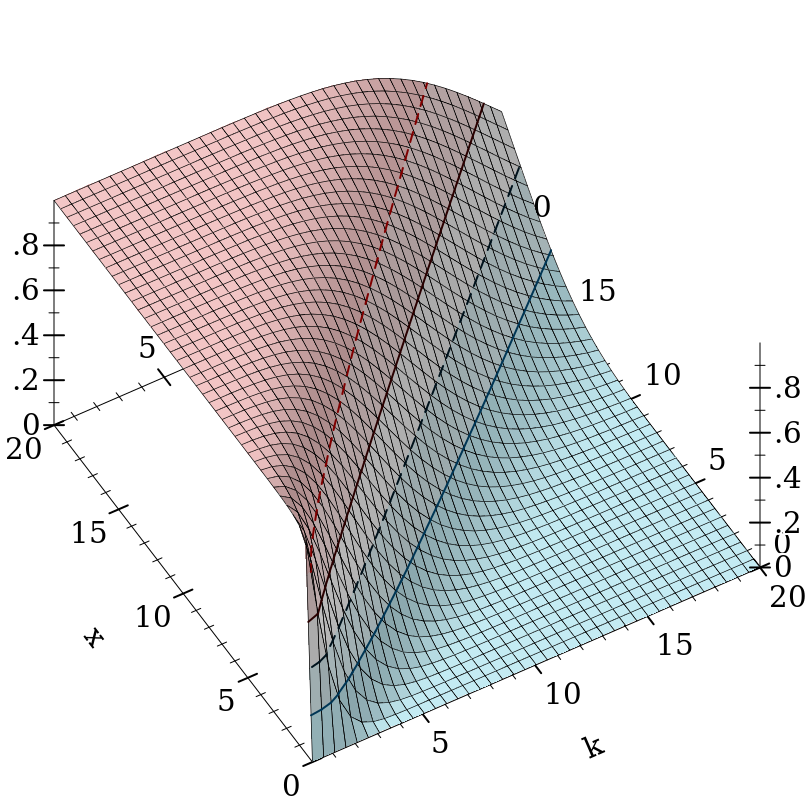> (gamma 4.0)

6.0

> (+ (gamma-inc 4.0 0.5 #f) (gamma-inc 4.0 0.5 #t))

6.0

> (gamma-inc 4.0 +inf.0)

6.0

> (/ (gamma-inc 200.0 50.0 #f) (gamma 200.0))

+nan.0

> (gamma-inc 200.0 50.0 #f #t)

2.0247590148473565e-57

> (gamma-inc 0 5.0)

gamma-inc: contract violation

expected: Positive-Real

given: 0

argument position: 1st

other arguments...:

5.0

> (gamma-inc 0.0 5.0)

+inf.0

 procedure(log-gamma-inc k x [upper? regularized?]) → Flonum k : Real x : Real upper? : Any = #f regularized? : Any = #f
Like (log (gamma-inc k x upper? regularized?)), but more accurate and without unnecessary overflow.

 procedure(beta-inc a b x [upper? regularized?]) → Flonum a : Real b : Real x : Real upper? : Any = #f regularized? : Any = #f
Computes the incomplete beta integral for a > 0, b > 0 and 0 <= x <= 1. When upper? = #f, it integrates from zero to x; otherwise, it integrates from x to one.

If you are doing statistical work, you should probably use beta-dist instead, which is defined in terms of beta-inc and is more flexible (e.g. it allows negative x).

Similar identities should hold as with gamma-inc.

Example:
 > (plot3d (isosurfaces3d (λ (a b x) (beta-inc a b x #f #t)) 0.1 2.5 0.1 2.5 0 1 #:label "beta(a,b,x)") #:x-label "a" #:y-label "b" #:z-label "x" #:angle 20 #:altitude 20 #:legend-anchor 'top)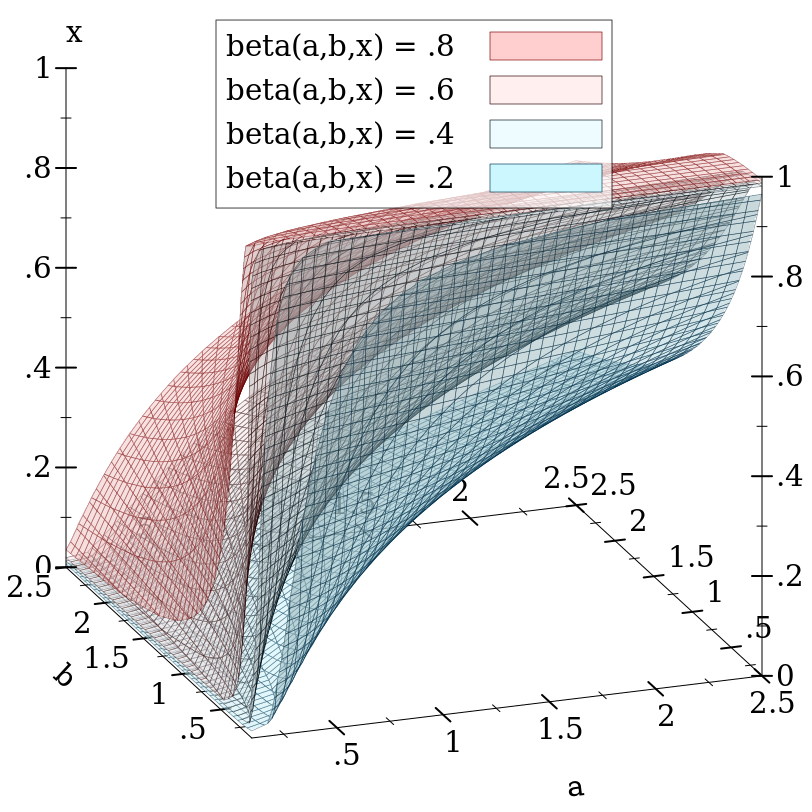procedure(log-beta-inc a b x [upper? regularized?]) → Flonum a : Real b : Real x : Real upper? : Any = #f regularized? : Any = #f
Like (log (beta-inc a b x upper? regularized?)), but more accurate and without unnecessary overflow.

While most areas of this function have error less than 5e-15, when a and b have very dissimilar magnitudes (e.g. 1e-16 and 1e+16), it exhibits catastrophic cancellation. We are working on it.

 procedure(Fresnel-S x) → Real x : Real
 procedure(Fresnel-C x) → Real x : Real
 procedure(Fresnel-RS x) → Real x : Real
 procedure(Fresnel-RC x) → Real x : Real
Compute the Fresnel integrals. Where
The first two are sometimes also referred to as the natural Fresnel integrals.

Examples:
 > (plot (list (function Fresnel-RS 0 5 #:label "Fresnel-RS(x)") (function Fresnel-RC 0 5 #:color 2 #:label "Fresnel-RC(x)")))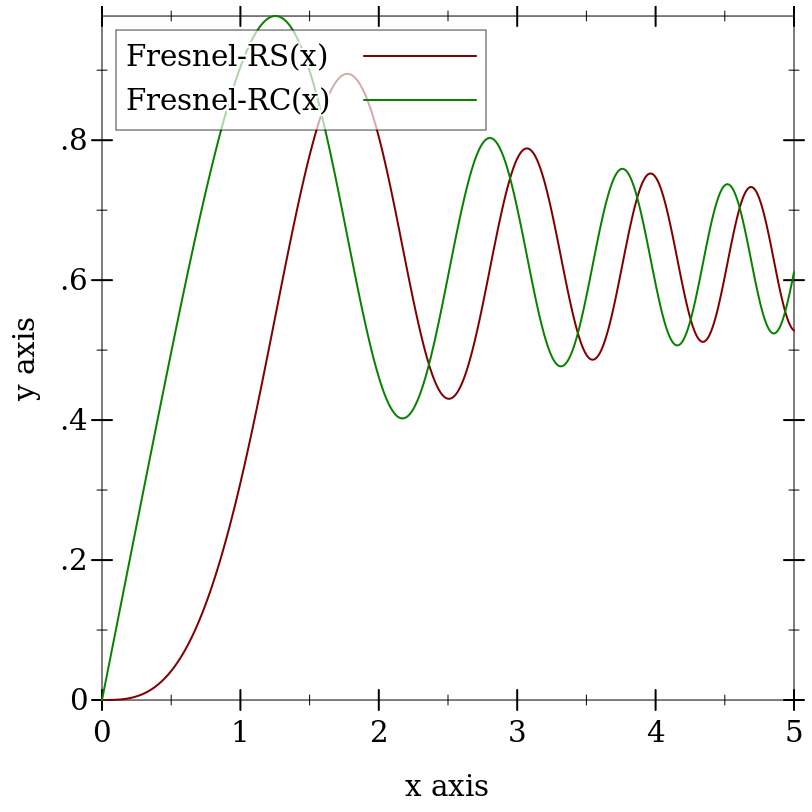> (plot (parametric (λ (t) (list (Fresnel-C t) (Fresnel-S t))) -5 5 #:label "Euler spiral"))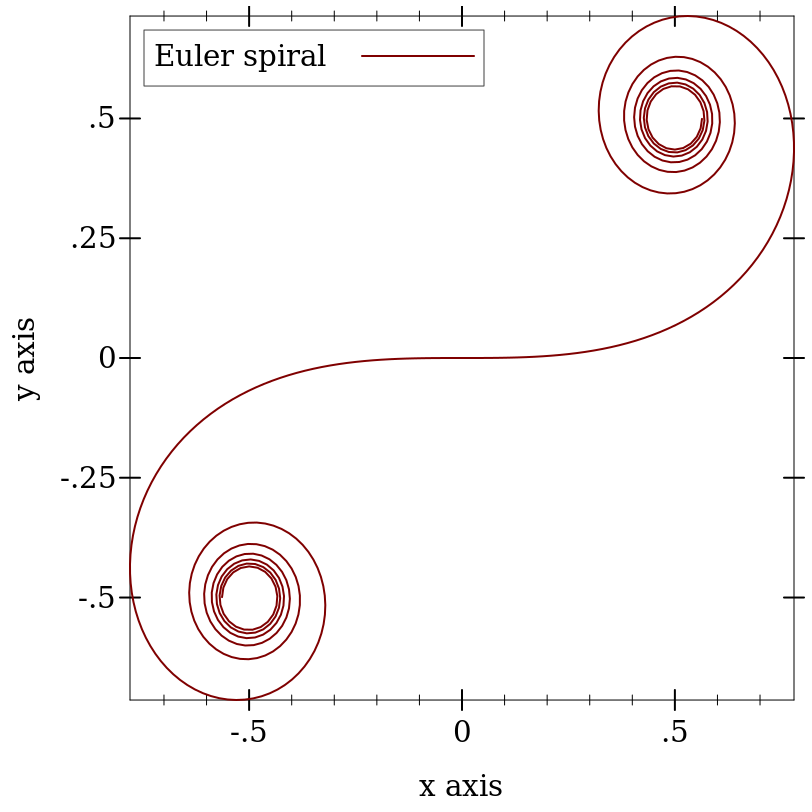> (Fresnel-RS 1)

0.31026830172338116

> (* (sqrt (/ pi 2)) (Fresnel-S (* (sqrt (/ 2 pi)) 1)))

0.31026830172338116

Spot-checks within the region 0<=x<=150 sugest that the error is no greater than 1e-14 everywhere that has been tested, and usually is lower than 2e-15.

#### 3.2Flonum Functions 🔗 ℹ

 procedure(flgamma x) → Flonum x : Flonum
 procedure x : Flonum
 procedure(flpsi0 x) → Flonum x : Flonum
 procedure(flpsi m x) → Flonum m : Integer x : Flonum
 procedure(flerf x) → Flonum x : Flonum
 procedure(flerfc x) → Flonum x : Flonum
 procedure(fllambert x) → Flonum x : Flonum
 procedure(fllambert- x) → Flonum x : Flonum
 procedure(flzeta x) → Flonum x : Flonum
 procedure(fleta x) → Flonum x : Flonum
 procedure(flhurwitz-zeta s q) → Flonum s : Flonum q : Flonum
 procedure(flbeta x y) → Flonum x : Flonum y : Flonum
 procedure(fllog-beta x y) → Flonum x : Flonum y : Flonum
 procedure(flgamma-inc k x upper? regularized?) → Flonum k : Flonum x : Flonum upper? : Any regularized? : Any
 procedure(fllog-gamma-inc k x upper? regularized?) → Flonum k : Flonum x : Flonum upper? : Any regularized? : Any
 procedure(flbeta-inc a b x upper? regularized?) → Flonum a : Flonum b : Flonum x : Flonum upper? : Any regularized? : Any
 procedure(fllog-beta-inc a b x upper? regularized?) → Flonum a : Flonum b : Flonum x : Flonum upper? : Any regularized? : Any
 procedure x : Flonum
 procedure x : Flonum
Flonum versions of the above functions. These return +nan.0 instead of raising errors and do not have optional arguments. They can be a little faster to apply because they check fewer special cases.